Luo, P., Xin, C., Ma, X., Liang, Y., Jiao, Z., Xu, S., and Zhang, J. (2013). "Process modeling of the batch acetylation of cotton linter pulp," BioRes. 8(4), 5635-5643.

#### Abstract

Following previous kinetic investigations of the acetylation of cotton linter pulp (Luo et al. 2013), a mathematical model was set up based on the mass and energy balances to simulate the batch acetylation process; a particular goal was to predict the temperature profile. The equations were discretized using a time-dependent finite difference method. The parameters for the model, including the kinetic parameters and heat transfer rate, were well estimated from the literature and our previous work. The model using the least-mean-square-error criterion optimizes the unknown parameters. The proposed model provides an accurate prediction of the process, including the temperature profile, peak temperature, and DS value under the peak temperature. The content of the catalyst, sulfuric acid, has a dramatic effect on the temperature profile. A slight increase in sulfuric acid content will lead to a faster dissolution process for the sulfated cellulose fibers, whereas the reaction rate of the sulfated fibers with acetic anhydride in the liquid phase is not affected. The optimized values of the activation energy are 11.0 and 7.6 kJ/mol for the dissolution processes with low and high sulfuric acid contents, respectively.

Process Modeling of the Batch Acetylation of Cotton Linter Pulp

Peicheng Luo,a,* Chuanxian Xin,a Xiaolong Ma,b,* Yinchun Liang,Zhen Jiao,a Songwei Xu,b and Jie Zhang b

Following previous kinetic investigations of the acetylation of cotton linter pulp (Luo et al. 2013), a mathematical model was set up based on the mass and energy balances to simulate the batch acetylation process; a particular goal was to predict the temperature profile. The equations were discretized using a time-dependent finite difference method. The parameters for the model, including the kinetic parameters and heat transfer rate, were well estimated from the literature and our previous work. The model using the least-mean-square-error criterion optimizes the unknown parameters. The proposed model provides an accurate prediction of the process, including the temperature profile, peak temperature, and DS value under the peak temperature. The content of the catalyst, sulfuric acid, has a dramatic effect on the temperature profile. A slight increase in sulfuric acid content will lead to a faster dissolution process for the sulfated cellulose fibers, whereas the reaction rate of the sulfated fibers with acetic anhydride in the liquid phase is not affected. The optimized values of the activation energy are 11.0 and 7.6 kJ/mol for the dissolution processes with low and high sulfuric acid contents, respectively.

Keywords: Process modeling; Batch acetylation; Cotton linter; Cellulose acetate

Contact information: a: School of Chemistry and Chemical Engineering, Southeast University, Nanjing 211189, China; b: Nantong Cellulose Fibers Co., Ltd., Nantong 226008, China;

* Corresponding authors: luopeicheng@seu.edu.cn; mxl@ncfcinfo.com

INTRODUCTION

Commercial acetylation of cellulose (CE) has been carried out almost exclusively with sulfuric acid catalyst, in which sulfuric acid reacts with solid fibers, creating a complicated multiphase reaction system. Although this process has been commercialized successfully since the 1940s (Malm et al. 1946), many researchers are still investigating it due to the complicated mechanism (Steinmeier 2004; Tang et al. 1997; Yang et al. 2008). Moreover, finding alternative sources of cellulose fibers instead of fibers from wood pulp, especially those using substitute raw materials (e.g., cotton linter, crop straw, rice husk, and wood chip), has called for special investigation on the particular cellulose source. For instance, Cerqueira et al.(2007) optimized the acetylation process of waste sugarcane bagasse cellulose catalyzed by sulfuric acid.

Generally, the classical commercial process consists of two steps: acetylation followed by hydrolysis of the tri-ester, in which acetylation plays a more important role. The acetylation procedure involves complicated acetylation reactions of primary and secondary hydroxyl groups on the cellulose chains and rapid hydrolysis reaction of acetic anhydride with the moisture in cellulose fibers, as well as the physical melting process of the frozen mixed acid crystals. The melting process is endothermic, whereas the hydrolysis of acetic anhydride and acetylation reactions are exothermic, which causes a complicated temperature change. Because the performance of the final product is greatly affected by the temperature profile of the process, a coolant (e.g., methylene chloride) is passed through the external jacket of the stirred vessel to regulate the temperature during the whole process. Thus, it is necessary to give a precise energy balance involving heat transfer, melting of the mixed acid crystals, and the enthalpy of the multiple reactions. To provide instruction for the control of an industrial plant, Dubey et al. (2006) constructed a mathematical model based on support vector regression to predict the temperature profile of the process. However, in addition to the complexity of their model, they did not report the detailed parameters of the model, including the kinetic parameters, for proprietary reasons.

In the previous work (Luo et al. 2013), the kinetic aspects of the acetylation process of cotton linter pulp were investigated based on the assumptions made by Sassi and Chanzy (1995). The dissolution of the sulfated layers or chains is considered, i.e., when the cellulose chains on the surface of the solid fibers are sufficiently sulfated, they will become soluble and move from the crystal into the reaction medium. Then, some new surface is exposed for subsequent reactions. The multiple acetylation reactions and the dissolution process are all described by Arrhenius expressions with kinetic parameters estimated by non-linear regression. In this paper, following our previous work, we focus on modeling the whole acetylation process of cotton linter pulp; in particular, the goal was to predict the temperature profile of the process, on the basis of the previously reported kinetic parameters. This model also considers the hydrolysis of acetic anhydride, the melting of the frozen mixed acid crystals, and heat transfer.

COMMERCIAL ACETYLATION PROCESS

The acetylation process was carried out on an industrial plant. Cotton linter pulp was used as the source of cellulose fibers. The moisture content of the pulp was about 7%. The pulp was then shredded in an industrial shredder to make it fluffy, yielding fibers with an average value of 19.0 μm in diameter and 1495 μm in length. The morphology pictures of the pulp and the method of size measurement was given in a previous article (Luo et al. 2013).

The commercial process studied in this paper is the so-called ‘acetic acid process’, i.e. acetic acid acts as swelling reagent and cellulose triacetate can be solubilized in the reaction medium as it is being produced. A ~6.5 m3 batch stirred vessel with an external jacket was used as the acetylation reactor. First, shredded pulp was pretreated for about 1 hour by adding a certain amount of acetic acid to activate the fibers. Then, the activated cellulose stream and a stream in a partially frozen state, consisting of sulfuric acid, acetic anhydride, and acetic acid, were added to the stirred vessel sequentially. When in contact with moisture, acetic anhydride undergoes rapid hydrolysis, releasing significant amounts of heat, which subsequently causes the melting of the frozen mixed acid crystals. Meanwhile, the complicated acetylation reactions occur when sufficient liquid sulfuric acid is present in the system. The reaction temperature was regulated by varying the flow rate of the coolant in the external jacket of the vessel. When the ‘peak temperature’ of the process came, the coolant was then shut down and a further ~20 min reaction was carried out to make thorough acetylation reactions as far as possible. It is noted that detailed feed compositions and operation conditions are not listed in this study for proprietary reasons.

MODEL DESCRIPTION

Basis for the Model

The model for the batch acetylation process in a stirred vessel has been developed using the mass and energy balances. The system in the vessel is regarded as a pseudo-homogeneous “solution”, i.e., the unreacted solid fibers and mixed solid acid crystals are all treated as similar species.

The melting of the mixed acid to the liquid phase and the dissolution of the sulfated fiber chains are regarded as “chemical reactions”. Thus, the mass balance equation for all species in the system can be written as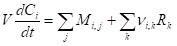, (1)

where V is the reaction volume in the system, Ci represents the mass concentration of the ith species, and Mi,j represents the mass flow rates of that species in the jth streams. Rk is the mass reaction rate with the stoichiometric coefficient of the species, υi,k, in the kth reaction.

Note that the melting rate of mixed acid crystals is also present in the terms of the above reaction rate. The energy balance in the batch reactor can be written as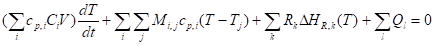, (2)

where cp,i is the mass specific heat capacity of the species and Tj and T are the temperature of the jth stream and the average temperature in the batch reactor, respectively. ∆HR,k(T) represents the enthalpy of the kth reaction, including the melting of the mixed acid crystals to the liquid phase at temperature T. The term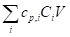stands for the total heat capacity of the system. Ql represents all the other heat sources, which includes the heat transfer to the system in the external jacket and energy loss to the environment.

Methodology

Figure 1 shows a schematic methodology for solving the model. The equations of mass and energy balances are discretized using a time-dependent finite difference method. The model is implemented in Matlab®. The approaches for the model parameters are listed in the next section.

For those unknown parameters, e.g.U1U2, and U3, a series of values are assigned in a reasonable range. The model then predicts the acetylation process, in particular, the temperature profile, given these parameters. Two commercial acetylation processes are selected to calculate the mean square error between the numerical predictions and the results from commercial processes. Finally, the least-mean-square-error criterion is used to obtain the optimized parameters for the model.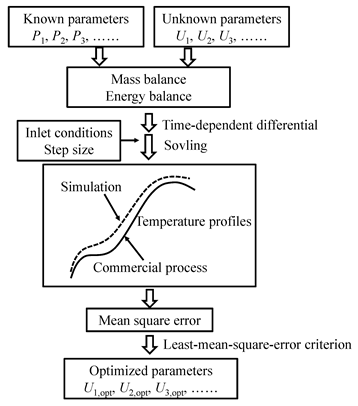Fig. 1. Methodology for the model predictions

RESULTS AND DISCUSSION

Approaches for the Model Parameters

The initial mass concentration of all species in each stream transferred to the vessel is not illustrated in this study for proprietary reasons. The physical properties, including mass specific heat capacity and density of water, sulfuric acid, acetic anhydride, cellulose, and cellulose acetate, are estimated by the corresponding correlations reported in Ullmann’s Encyclopedia of Industrial Chemistry. The other kinetic parameters are estimated as follows.

At the initial stage of the process, because there is no liquid sulfuric acid in the system, the acetylation of cellulose is comparatively slow. The main reaction is the hydrolysis of acetic anhydride with the moisture in the pulp, which is a strong exothermic reaction and leads to a sharp increase in the temperature during the initial several minutes. The hydrolysis of acetic anhydride (Ac2O) reaction, which produces acetic acid (AcOH), can be represented as

Ac2O＋H2O→2AcOH (3)

Theoretically, the apparent rate of reaction (3) is determined by both the intrinsic rate of the reaction and the transport rate of water from the solid cellulose fibers to the liquid phase. However, the cellulose pulp is pretreated with liquid acetic acid before being transferred to the vessel in the commercial process. Thus, the water in the solid fibers has been extracted into the liquid phase of acetic acid effectively. When the mixed acid crystals are added to the system, the majority of water is indeed in the liquid phase. In the present study, reaction (3) is considered to be a pseudo-homogeneous reaction in concentrated acetic acid. The reaction rate can be written as (Asprey et al. 1996)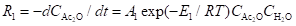, (4)

where A1 and E1 are pre-exponential factor and the activation energy of reaction (3), respectively. R is the gas constant. For the kinetic parameters of the hydrolysis reaction, Janssen et al.(1957) studied the kinetic rate in concentrated acetic acid. They found that this reaction closely conformed to the bimolecular law. Considering that the system in this work is similar to the one Janssen et al. (1957) investigated, the kinetic parameters are assigned from their work (see Table 1). The enthalpy of the reaction is 3300 kJ/kg water.

Table 1. Values for the Model Parameters from Literatures and the Present Model with Optimized Values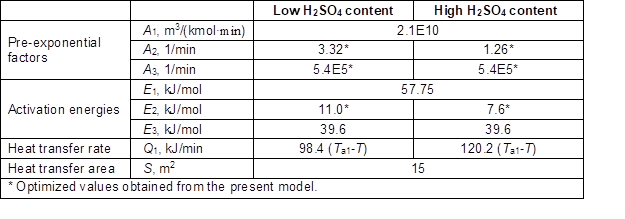For the melting process of the mixed acid crystals, it is difficult to give a precise rate-dependent equation because it occurs in a complicated reaction medium. Experi-mental techniques, e.g., differential scanning calorimetry (DSC), are alternative methods for the study of the phase-changing behavior in the complicated system. In previous work (Liang et al. 2008), the authors obtained the scaling profile of the melting enthalpy vs. temperature by DSC measurement under the conditions of the commercial process. Thus, the melting rate of the mixed acid crystals can be represented as a function of the system temperature.

In the previous study (Luo et al. 2013), the acetylation process consisted of the dissolution of sulfated-cellulose (Sulf-CE) chains into the liquid phase and the further reactions of Sulf-CE with Ac2O, which produce the final product, cellulose acetate (CA). Thus, the two consecutive steps can be written as: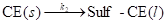, (5)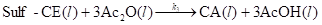. (6)

Because acetic anhydride is present in excess, the acetylation reaction can be regarded as a pseudo first-order reaction. Thus, the dissolution rate of equation (5), and the acetylation reaction rate of equation (6), can be written in Arrhenius expressions as follows,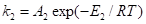, (7)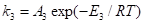, (8)

where A2 and A3 are pre-exponential factors and E2 and E3 are the activation energies of reactions (5) and (6), respectively. The enthalpy of the acetylation reaction (6) is adopted from the experimental results of Liang et al. (2010). Considering that the activation energy of the acetylation reaction in the liquid phase is only determined by the intrinsic characteristics, the value of E3 is assigned from the authors’ previous study (Luo et al. 2013). However, the dissolution process is determined by the sulfuric acid content in the system, E2, and the pre-exponential factors are estimated with optimized values.

Heat Transfer Estimation

In the industrial process, the heat of the system is removed by coolant in the external jacket of the vessel. The heat transfer rate between the reaction system and coolant, Q1, is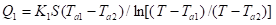, (9)

where K1 is the overall coefficient of heat transfer and S denotes the effective heat transfer area. TTa1, and Ta2 are the temperatures of reaction system, the inlet, and the outlet of the coolant, respectively. The heat transfer rate can also be written in terms of the enthalpy change of the coolant,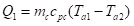, (10)

where mc is the mass flow rate of the coolant and cpc is the mass specific heat capacity of the coolant. Combining equations (9) and (10), one can obtain the following heat transfer rate equation using the parameters of Ta1T, and mc.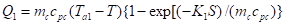. (11)

The term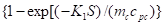in Equation 11 can be measured from the industrial reactor vessel with a similar reaction system without sulfuric acid, i.e., no reactions occur in the system. Thus, the temperature, T, was varied and the quantities mc and Ta1 were measured. This made it possible to estimate the term under different temperatures using Equations 10 and 11. The mathematical equations for heat transfer rate of two commercial processes are listed in Table 1.

When the system reaches the peak temperature, the coolant is shut down. Then, the heat is transferred from the system to the quiescent coolant in the vessel jacket. Considering that the convective heat transfer coefficient is much higher than the conductive heat transfer coefficient at the side of the coolant in the vessel jacket, the overall coefficient of heat transfer can be approximately written as Equation 12,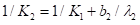, (12)

where b2 is the width of the jacket flow channel and λ2 is the heat conductivity of the coolant. The corresponding heat transfer rate is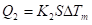, (13)

where ∆Tm is the logarithmic mean temperature difference between the temperature of the system and that of the quiescent coolant in the vessel jacket.

Temperature Profile Predictions

As stated above, only 3 parameters, A2A3, and E2, are not assigned. Following the methodology illustrated in Fig. 1, the values of these three parameters are varied and the corresponding mean-square-error of the temperature profile is calculated for two commercial processes. The temperature profiles obtained with the optimized parameters are plotted in Fig. 2. It can be seen that the predicted profiles agree well with those from the commercial processes. Moreover, in a typical commercial process, the degree of substitution, DS, always has a value of 2.7~2.9 when the peak temperature occurs. In this study, the predicted values of DS for two processes are 2.77 (high H2SO4 content) and 2.81 (low H2SO4 content). This is a further indication of the accuracy of the proposed model.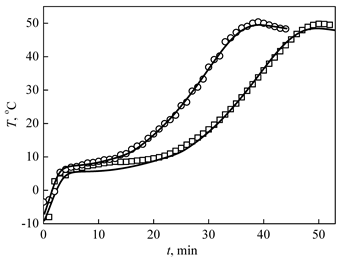Fig. 2. Temperature profiles from the model (solid lines) and two commercial processes with different H2SO4 contents. (□) low H2SO4 content; (○) 13.5% increase in H2SO4 content compared with the process represented by (□).

Effect of the Sulfuric Acid Content

From Fig. 2, it can be observed that sulfuric acid has a dramatic effect on the acetylation process, i.e., a 13.5% increase in H2SO4 content will lead to a faster acetylation process. For example, it takes 39.2 min to come to the peak temperature with a high H2SO4 content, whereas it takes 49.7 min for the process with a low H2SO4 content. As stated in the previous study by the authors (Luo et al. 2013), H2SO4 plays an important role in the dissolution of the sulfated cellulose fibers, whereas it has little effect on the kinetic aspects of the acetylation reactions in the liquid phase. This can be confirmed by comparing the optimized kinetic parameters of Equations 7 and 8. In this study, the optimized pre-exponential factors of the acetylation reactions for the two commercial processes all have values of 5.4×105 1/min, i.e., as expected, H2SO4 content has no effect on the reaction rate of acetylation reactions in the liquid phase. However, the optimized values of the activation energy of the dissolution process are 11.0 and 7.6 kJ/mol for the processes with low and high H2SO4 contents, respectively. In other words, it is helpful to accelerate the dissolution of the sulfated cellulose fibers with a high H2SO4 content, which is also in accordance with the aspects reported in the literature (Araki 1952; Malm et al. 1961).

CONCLUSIONS

1. A mathematical model based on the mass and energy balances for the batch acetylation process of cotton linter pulp has been proposed. The equations are discretized using a time-dependent finite difference method. The parameters for the model, including the kinetic parameters and heat transfer process, are well estimated from the literature and previous work by the authors. The model using the least-mean-square-error criterion optimizes the unknown parameters.
2. The proposed model provides an accurate prediction of the batch acetylation process, in particular, the temperature profile, peak temperature, and DS value under the peak temperature.
3. The catalyst, sulfuric acid, has a dramatic effect on the temperature profile. A slight increase in sulfuric acid content will lead to a faster acetylation process, especially to quicken the dissolution process of the sulfated cellulose fibers. The optimized values of the activation energy are 11.0 and 7.6 kJ/mol for dissolution processes with low and high H2SO4contents, respectively.

ACKNOWLEDGMENTS

The authors are grateful for the financial support of Nantong Cellulose Fibers Co., Ltd. We also acknowledge financial support from the National Natural Science Foundation of China (Grant No. 21006011) and the Natural Science Foundation of Jiangsu Province (Grant No. BK2010410).

REFERENCES CITED

Araki, T. (1952). “Studies on cellulose acetate. 3. Combination of sulfuric acid during the acetylation of cellulose and the mechanism of acetylation,” Textile Research Journal 22(10), 630-636.

Asprey, S. P., Wojciechowski, B. W., Rice, N. M., and Dorcas, A. (1996). “Applications of temperature scanning in kinetic investigations: The hydrolysis of acetic anhydride,” Chemical Engineering Science 51(20), 4681-4692.

Cerqueira, D. A., Rodrigues, G., and Meireles, C. D. (2007). “Optimization of sugarcane bagasse cellulose acetylation,” Carbohydrate Polymers 69(3), 579-582.

Dubey, A., Realff, M. J., Lee, J. H., Schork, F. J., Butte, A., Olle, B., and Kizer, L. E. (2006). “Modeling and inferential control of the batch acetylation of cellulose,” AIChE Journal 52(6), 2149-2160.

Janssen, H. J., Haydel, C. H., and Greathouse, L. H. (1957). “Hydrolysis of acetic anhydride in concentrated acetic acid without catalysis,” Industrial and Engineering Chemistry 49(2), 197-201.

Liang, Y., Gu, H., Guo, Y., Cao, J., and Zhang, J. (2008). “Study on the phase-changing behavior of mixed acid in the manufacture of cellulose acetate by dsc,” Synthetic Technlogy and Application (Chinese) 23(4), 47-49.

Liang, Y., Ma, X., Chen, Y., Huang, Y., Cao, J., and Zhang, J. (2010). “Study on the acetylation reaction of cotton linters by reaction calorimeter,” Journal of Cellulose Science and Technology (Chinese) 18(2), 38-43.

Luo, P., Cao, C., Liang, Y., Ma, X., Xin, C., Jiao, Z., Cao, J., and Zhang, J. (2013). “Kinetic study of the acetylation of cotton linter pulp,” BioResources 8(2), 2708-2718.

Malm, C. J., Tanghe, L. J., and Laird, B. C. (1946). “Preparation of cellulose acetate – action of sulfuric acid,” Industrial and Engineering Chemistry 38(1), 77-82.

Malm, C. J., Tanghe, L. J., and Schmitt, J. T. (1961). “Catalysts for acetylation of cellulose,” Industrial and Engineering Chemistry 53(5), 363-367.

Sassi, J. F., and Chanzy, H. (1995). “Ultrastructural aspects of the acetylation of cellulose,” Cellulose 2(2), 111-127.

Steinmeier, H. (2004). “Chemistry of cellulose acetylation,” Macromolecular Symposia 208, 49-60.

Tang, L. G., Hon, D. N. S., and Zhu, Y. Q. (1997). “An investigation in solution acetylation of cellulose by microscopic techniques,” Journal of Applied Polymer Science 64(10), 1953-1960.

Yang, Z. P., Xu, S. W., Ma, X. L., and Wang, S. Y. (2008). “Characterization and acetylation behavior of bamboo pulp,” Wood Science and Technology 42(8), 621-632.

Article submitted: July 1, 2013; Peer review completed: Sept. 1, 2013; Revised version received and accepted: Sept. 15, 2013; Published: September 23, 2013.